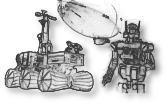## optimization/roboptim-core

Optimization for robotics: common API to various solvers
Directory:
optimization/roboptim-core (package's history)
Package version:
roboptim-core-3.1r2
http://www.roboptim.net
gnu-lgpl-v3
Source archive:
Description:
roboptim is a C++ generic non-linear optimization library for robotics composed of 3 levels: - core - posture - trajectory The core level allows users to define non-linear optimization problems in a generic way in order to use different back-ends. Currently the supported solvers are * cfsqp * ipopt A non-linear optimization problem can be expressed as follows: min f(x), x in R^n, Ce(x) = 0, Ci(x)<= 0 where - f is a scalar mapping defined over R^n, - Ce (equality constraints) is a mapping from R^n to R^m, - Ci (inequality constraint) is a mapping from R^n to R^p.
Run dependencies:
boost-libs>=1.34.1, boost-libs>=1.34.1, boost-libs>=1.34.1, boost-libs>=1.34.1, libstdc++, libtool-ltdl>=1.5.22, log4cxx>=0.10
Build dependencies:
boost-headers>=1.34.1, cmake>=2.6, digest>=20080510, doxygen>=1.5, eigen3>=3.2.0, g++>=4.6, gcc>=3, gm4>=1.4, latex>=3.14, libtool>=1.5.22, patch>=2.0, pax, pkg-config>=0.22, pkg_install>=20110805.12, tnftp>=20130505~ssl
General options:
debug
Produce debugging information for binary programs
c++-compiler alternatives:
g++
Use the GNU C++ compiler
clang++
Use the LLVM C++ compiler
ccache-g++
Use ccache and the GNU C++ compiler
ccache-clang++
Use ccache and the LLVM C++ compiler
c-compiler alternatives:
gcc
Use the GNU C compiler
clang
Use the LLVM C compiler
ccache-gcc
Use ccache and the GNU C compiler
ccache-clang
Use ccache and the LLVM C compiler